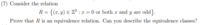# Proving an equivalence relation and finding equivalence classes

#### Cactusguy

##### New memberI feel like I'm just being stupid and not seeing this problem properly, but I can't figure out how to do this question.

I know an equivalence relation is when a relation is transitive, reflexive, and symmetric.
Reflexive: I know this is true, but I'm not sure how to prove it in proper terms.
"When x is odd, and y=x, xRy because y must be odd as well, and when x=0, y=x, xRy. Thus, xRx."
Is that how you do it?

Symmetric: If xRy and x is odd, then y must be odd as well. Thus, yRx.
But what if x=0? Can't y be anything when x is zero, thus it isn't symmetric?

Transitive: Not sure how to prove this one either.

Equivalence class: I know that an equivalence class is the set of all numbers x in A so that for any given a, xRa. How do I find this for this problem?

Thanks in advance, I have an exam tomorrow so I could really use the help. This is from my study guide.

#### ksdhart2

##### Senior Member
Symmetric: If xRy and x is odd, then y must be odd as well. Thus, yRx.
But what if x=0? Can't y be anything when x is zero, thus it isn't symmetric?
I agree with your assessment here. One need only provide a single counterexample to demonstrate that a relation is not symmetric. In this case, $$(0,2) \in R$$ but $$(2,0) \notin R$$, so R is not symmetric.

•Cactusguy

#### pka

##### Elite Member
Cactusguy, are you absolutely sure that you have written the exact instructions?
As presented this must mean that it is asking to show that $$\displaystyle \mathcal{R}$$ is an equivalence on the set $$\displaystyle \mathbb{Z}$$
However, $$\displaystyle 2\in\mathbb{Z}$$ BUT $$\displaystyle (2,2)\notin\mathcal{R}$$. Therefore $$\displaystyle \mathcal{R}$$ is not reflexive and cannot be an equivalence relation.

#### Cactusguy

##### New member
Yes, I copied the instructions directly from the study guide, that is exactly how the question is written. As ksdhart2 wrote above, it seems that the relation is not symmetric, so there must be an error in the problem or something.

However, you are right, it seems (2,2) proves that it is not reflexive. There's nothing specifying that x has to be odd or zero, right?

#### Cactusguy

##### New member
UPDATE: I emailed my professor about this, and he wrote back saying that the problem should not say x=0, but rather x=y.

So this is definitely an equivalence relation, now back to my original question, how do I going about proving it, and what is the equivalence class?

#### Cactusguy

##### New member
Reflexive: If y=x, then xRy, thus xRx.
Symmetric: When x is odd and xRy, then y must be odd as well. Therefore yRx. When x=y, xRx so xRy.
Transitive: How do I prove this?

Equivalence class: {(x,y): x,y in Z, x=y} U {(x,y) : x=2m+1, m in Z : y=2n+1, n in Z)}
Is that right?

#### pka

##### Elite Member
UPDATE: I emailed my professor about this, and he wrote back saying that the problem should not say x=0, but rather x=y. So this is definitely an equivalence relation, now back to my original question, how do I going about proving it, and what is the equivalence class?
So $$\displaystyle \mathcal{R}\subset ( \mathbb{Z}\times\mathbb{Z})$$ where $$\displaystyle (x,y)\in\mathcal{R}\iff x=y \text{ OR both of }x~\&~y\text{ are odd .}$$
Well agree that being reflexive & symmetric are obvious. If you have questions there, post exactly where the difficulty is.
Suppose that $$\displaystyle (x,y)~\&~(y,z)$$ are two points in $$\displaystyle \mathcal{R}$$.
If $$\displaystyle x=y$$ then $$\displaystyle x~\&~z$$ are both odd. Think about why that must be the case.
If $$\displaystyle x\ne y$$ then $$\displaystyle x~,y ~\&~z$$ are all odd. Think about why that must be the case.

If $$\displaystyle (1,1)\in\mathcal{R}~\&~(1,5)\in\mathcal{R} \Rightarrow (1,5)\in\mathcal{R}$$
If $$\displaystyle (3,1)\in\mathcal{R}~\&~(1,5)\in\mathcal{R} \Rightarrow (3,5)\in\mathcal{R}$$ HINT: all odd